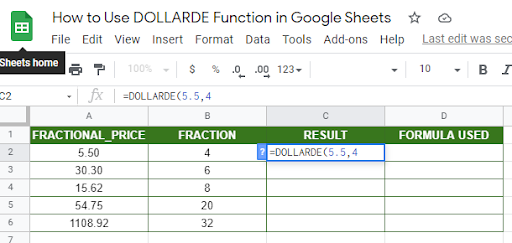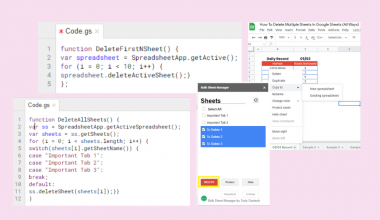# How to Use DOLLARDE Function in Google Sheets

The DOLLARDE function in Google Sheets is useful if you want to convert a price given as a decimal fraction into a decimal value, which is used in securities denominated in dollars.

The DOLLARDE function is the complete opposite of the DOLLARFR function. It converts a decimal fraction to its equivalent in decimal numbers. The DOLLARDE is another financial function mostly used by financial analysts who deal with financial reports and stock market quotes.

The rules for using DOLLARDE function in Google Sheets are as follows:

• The return values in DOLLARDE function can be used in other calculations or in charts.
• The DOLLARDE can also be used to convert non-dollar data.
• The DOLLARDE function accepts cell addresses as arguments.

Let’s take an example.

In baseball, since a pitcher needs 3 outs to complete one inning, the innings a pitcher throws are counted in thirds. This means that 12 innings and 2 outs, is not shown as 12.667, but instead as 12.2. Furthermore, 1 more out and a pitcher will have 13 innings pitched, instead of 8. Please see the given data below:To be able to get the total innings pitched of a specific baseball player, the values in Column B need to be converted to Decimal notation or format.

Values in Column B cannot be used to get the total innings pitched since it will yield the value 78.8, which isn’t a valid inning pitched number (78 innings and 8 outs).That’s where we can use the DOLLARDE function. We can convert the values in Column B, which we can use to get the total in Decimal notation. In this case, we used the SUM function to get the total innings pitched. See Column D below:In reverse, the DOLLARFR function can be used to the value 80.6667 in cell D9 to yield the total innings pitched 80.2, which means 80 innings and 2 outs.

Let’s have another example!

John wants to easily record time in Decimal format (i.e. 5.2, 6.3, 7.5). However, he likes it in a way that the Google Sheet reads as 5 hours and 20 mins, 6 hours and 30 mins and 7 hours and 50 mins, so that he can compute the total hours worked in Decimal format.John here used the DOLLARDE function in Column C to be able to convert the values in Column B into decimal hour format. Please refer to the formulas in Column D.

There are other ways that these example cases can be solved. But, the DOLLARDE function can surely be one of them if you’re looking for a quick solution.

Watch out for a more advanced tutorial and examples on how you can use DOLLARDE functions in the coming weeks. Be sure to subscribe to be notified.

Awesome! Let’s begin getting to know more about our DOLLARDE function in Google Sheets.

## The Anatomy of the DOLLARDE Function

So the syntax (the way we write) the DOLLARDE function is as follows:

`=DOLLARDE(fractional_price, fraction)`

Let’s dissect this thing and understand what each of these terms means:

• = the equal sign is just how we start any function in Google Sheets. It is how Google Sheets understand that we are asking it to either do a computation or use a function.
• DOLLARDE() this is our DOLLARDE function. DOLLARDE converts the given fractional notation into a decimal value. It will divide the fraction part of the value by the “fraction” specified by the user.
• fractional_price is the first argument that is a number expressed as an integer part and fraction part, separated by a decimal point.
• fraction is the second argument that is the integer (example 4, 8, or 32) to use in the denominator (divisor) of a fraction. It must be an integer. But if the decimal value is provided, Google Sheet will truncate it to an integer.

## A Real Example of Using DOLLARDE Function

Take a look at the example below to see how DOLLARDE functions are used in Google Sheets.As you can see, the DOLLARDE could change the entire value of the given decimal price in Column A.

Take a look at the example in cell A2, DOLLARDE function converts the fractional price number 5.50 to a number that reads as 5 and 5/4 or 5 + 5/4 in C2. 5/4 is equal to 1.25, which is then added to the integer 5 and gives us 6.25.For fractional price in cell A5, the function converts the number 54.75 to a number that reads as 54 and 75/20 or simply 54 + 75/20.You may make a copy of the spreadsheet using the link I have attached below.

## How to Use DOLLARDE Function in Google Sheets

1. Simply click on any cell to make it the active cell. For this guide, I will be selecting C2 where I want to show my result.1. Next, type the equal sign ‘=‘ to begin the function and then followed by the name of the function, which is our ‘dollar‘ (or ‘DOLLAR‘, not case sensitive).

1. You should find that the auto-suggest box appears with the names of the functions that all start with DOLLAR. You may use your mouse to click on the DOLLARDE or use your down arrow keys to highlight and hit the enter key. Alternatively, you may completely type DOLLARDE and hit Tab to let you use that function.1. Now the fun part! Let’s give our function the first argument, which is the decimal_price. You may pass a constant data by typing the exact decimal after the parenthesis.1. To let the Google sheet know that we’re done typing our first argument, we should now type in the delimiter or the character that separates each argument on a function. In this case, type comma(,) followed by the second argument, which is the fraction.1. Finally, just hit your Enter or Tab key. The active cell is now showing you the result or the return value of the DOLLARDE function.1. The result in C2 now reads as 5 and 5/4. You can check that if you divide 5 by 4, you will get 1.25, which is then added to the decimal (5) part of our given constant.1. Notice that in this example, we provided the exact value, constant, as the arguments of our DOLLARDE. Alternatively, these constants can be variable or simply cell addresses of your data.

1. Edit your function by changing the first and second argument into the cell addresses where your fractional_price and fraction are located. In this case, cell A2 and cell B2.1. Copy the formula down to the remaining rows.That’s pretty much it. You can now use DOLLARDE functions in Google Sheets together with the other numerous Google Sheets formulas to create even more powerful formulas that can make your life much easier.Our goal this year is to create lots of rich, bite-sized tutorials for Google Sheets users like you. If you liked this one, you'll love what we are working on! Readers receive ✨ early access ✨ to new content.

##### You May Also Like## How to Calculate Reverse Percentages in Google Sheets

This guide will show you how you can compute reverse percentages in Google Sheets using basic arithmetic operations.…## How To Delete Multiple Sheets In Google Sheets (All Ways)

Knowing how to delete multiple sheets in Google Sheets is useful if you want to get rid of…## How to Use T.DIST Function in Google Sheets

The T.DIST function in Google Sheets is useful when you need to return the Student distribution of a…## How to Use ARRAYFORMULA Function in Google Sheets

The ARRAYFORMULA function in Google Sheets is useful to apply a formula to an entire column in Google…